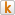# The Prediction Of Body Fat In Japanese Males Living In Australia

Por: Colin Binns, Deborah Kerr e Masaharu Kagawa.

Athens 2004: Pre-olympic CongressSend to Kindle

Introduction
Anthropometric equations used to predict body fat are known to be population specific. When using percentage body fat
equations, the equation should only be applied to populations of similar ethnic background to the original study
population. An equation used to predict body fat in Japanese subjects was developed 30 years ago , using
hydrodensitometry as the criterion method. This study aimed to develop and evaluate new Japanese-specific body fat
prediction equations as well as to determine the applicability of the Nagamine and Suzuki equation.

Methods
The body composition of 45 Japanese males (aged 18-40) living in Australia (JA) was obtained by whole-body
scanning using dual energy x-ray absorptiometry (DXA), and anthropometry using a standard protocol with
combinations of body density and body fat prediction equations . Limit of agreement analysis was conducted to
compare differences in results obtained from two methods. From the regression analysis, new %BF prediction equations
were proposed.

Results
Results from DXA were equivalent to the values obtained using the ‘Durnin and Womersley’ body density prediction
equation (Graph1). The results were significantly different (p<0.05) from the predicted %BF using the ‘Nagamine and
Suzuki’ body density prediction equation (Graph2). Among new regression equations proposed, the equation of y =
0.376 + 0.402*(abdominal) + 0.772*(medial calf) + 0.217*(age) showed the highest correlation (R2 = 0.786, SEE =
2.691) with the results obtained from DXA scans.

Conclusion
The %BF results obtained from anthropometry using the ‘Durnin and Womersley’ body density prediction equation
were comparable to DXA results for the Japanese males. However, the Japanese-specific ‘Nagamine and Suzuki’ body
density prediction equation did not predict %BF of Japanese males well. Using DXA and anthropometry results, new
%BF prediction equations were proposed. Further research is needed in a larger Japanese sample of both genders and
age range to assess the suitability of the proposed equation.

References
. Nagamine S, Suzuki S. (1964) Human Biology 36:8-15.
. Norton K, Olds T, (eds). (1996) Anthropometrica. Sydney: University of New South Wales Press.

NOTA: O texto com a iconografia está no anexo

Ver Arquivo (PDF)

### Comentários

:-)

© 1996-2020 Centro Esportivo Virtual - CEV.
O material veiculado neste site poderá ser livremente distribuído para fins não comerciais, segundo os termos da licença da Creative Commons.